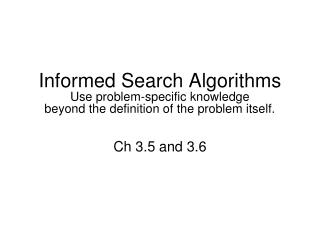DownloadDownload PresentationCh 3.5 and 3.6

# Ch 3.5 and 3.6

Download Presentation## Ch 3.5 and 3.6

- - - - - - - - - - - - - - - - - - - - - - - - - - - E N D - - - - - - - - - - - - - - - - - - - - - - - - - - -
##### Presentation Transcript

1. Informed Search AlgorithmsUse problem-specific knowledge beyond the definition of the problem itself. Ch 3.5 and 3.6

2. Outline • Heuristic Search Methods • Best-first search algorithms • Greedy best-first search • A* search • Dominance • Relaxed Problems

3. Heuristic Search Methods node to be expanded • Estimate of “desirability”. • Use an evaluation functionf(n) for each node • Evaluation function f(n) = f(h(n)) (heuristic) h(n)= estimate of cost from n to goal h(n)=0 if n is a goal node • Order the nodes in fringe in decreasing order of desirability. • Expand most desirable unexpanded node. • Focus on states estimated to be most desirable (i.e. states with lowest or highest heuristic values). • The performance highly depends on how good is its h(n). • A good heuristic function can often find good (though possibly non-optimal) solutions in less than exponential time.

4. Additional thoughts on H • People often are satisfied with a good, though not optimal, solutions. • Although heuristic search may not be better than uninformed search in worst cases, worst cases rarely arise in the real world. • Trying to understand why a heuristic works, or why it does not work, often leads to a deeper understanding of the problem. • There is a trade-off between the time spent in computing heuristic values and the time spent in search.

5. Greedy best-first search • Idea: expands the node that appears to be closest to goal. • Evaluation function f(n)=h(n) • e.g., h(n)=straight-line distance from n to Bucharest

6. Romania with step costs in km

7. Greedy best-first search example Comparing all nodes in fringe

8. Properties of greedy best-first search • Complete? • Time? • Space? • Optimal?

9. A* search • Ideas • Minimizing the total estimated solution cost. • Avoid expanding paths that are already expensive • Evaluation function f(n) = g(n) + h(n) • g(n) = cost to reach n from root • h(n) = cost from n to goal • f(n) = estimated total cost of path through n to goal

10. A* search example Comparing all nodes in fringe

11. A* search example Total cost =140+80+97+101 =418

12. BFS and DFS are special cases of greedy search. • Uniform-cost search are special cases of A*.

13. Admissible heuristics Theorem: If h(n) is admissible, A* using TREE-SEARCH is optimal. • A heuristic h(n) is admissible iff n h(n) ≤ h*(n), where h*(n) is the true cost to reach the goal state from n. • An admissible heuristic never overestimates the cost to reach the goal, i.e., it is optimistic. • Question: hSLD(n) is admissible?

14. Optimality of A* (proof) • Suppose some suboptimal goal G2has been generated and is in the fringe. Let n be an unexpanded node in the fringe such that n is on a shortest path to an optimal goal G. Idea: in this case, we will expand n not G2, i.e. we need to prove f(n)<f(G2).

15. Consistent heuristics Theorem: If h(n) is consistent, A* using GRAPH-SEARCH is optimal. • A heuristic is consistent if for every node n, every successor n' of n generated by any action a, h(n) ≤ c(n,a,n') + h(n') (triangle inequality) Idea: if h is consistent, f(n) is non-decreasing along any path, f(n') = g(n') + h(n') = g(n) + c(n,a,n') + h(n') ≥ g(n) + h(n) = f(n) So the first goal node selected for expansion must be optimal, since all later nodes will be at least as expensive. • hSLD(n) is consistent? • Every consistent heuristic is admissible. (Ex. 3.29).

16. Optimality of A* • A* expands nodes in order of increasing f value. • Gradually adds "f-contours" of nodes • Contour i has all nodes that fi < fi+1.

17. Properties of A* • Complete? Yes (unless there are infinitely many nodes with f ≤ f(G) ). • Time? Exponential. A* expands all nodes with f(n)<C*. • Space? Keeps all nodes in memory. • Optimal? Depends on quality of h. Yes, if h is admissible or consistent.

18. Example of Admissible heuristics Complexity of the problem in general Average b: 3. Average d (step): 22. State space: bd=322=31010 including repeated states. State space: 170,000 without repeated states.

19. Dominance • If h2(n) ≥ h1(n) for all n (both admissible) • then h2dominatesh1 • h2is better for search • f(n)<C* => h(n)<C*-g(n) • Typical search costs (average number of nodes expanded): • d=12 IDS = 3,644,035 nodes A*(h1) = 227 nodes A*(h2) = 73 nodes • d=24 IDS = too many nodes A*(h1) = 39,135 nodes A*(h2) = 1,641 nodes h1 h2 C*-g(n) Saving: (227-73)/227=67.8% (39135-1641)/39135=95.8%

20. Relaxed problems • A problem with fewer restrictions on the actions is called a relaxed problem. • The cost of an optimal solution to a relaxed problem is an admissible heuristic for the original problem. ≥

21. An 8-puzzle example • A tile can move A->B if • A is horizontally/vertically adjacent B. • B is blank. • Relaxed problem: • (1) A tile can move A->B. • (2) A tile can move A->B, if A is adjacent B. • (3) A tile can move A->B, if B is blank. • If the rules of the 8-puzzle are relaxed so that a tile can move anywhere (1), then h1(n) gives the shortest solution. • If the rules are relaxed so that a tile can move to any adjacent square (2), then h2(n) gives the shortest solution.